### Spencer R. Hall, Val H. Smith, David A. Lytle, and Mathew A. Leibold. 2005. Constraints on primary producer N:P stoichiometry along N:P supply ratio gradients. Ecology 86:1894–1904.

Appendix B. Mechanisms for N:P attenuation: chemostat models.

In this appendix we presented the model used to derive several explanations for deviations from a 1:1 relationship between N:P stoichiometry of producers and N:P supply ratios. Legovic and Cruzado (1997) and Klausmeier et al. (2004) present analytical results and stability analysis of very similar models, so we did not duplicate them here. We included these equations mainly to support the patterns shown in Fig. 4 of the article.

No Quota Saturation

In Fig. 4A, we showed results from a standard population dynamic model for plant carbon and nutrient quotas for N and P. These ordinary differential equations resembled those that have been used in other stoichiometrically explicit models (e.g., Andersen 1997, Grover 1997, Geider et al. 1998) and were almost identical to Legovic and Cruzado's (1997) and Klausmeier et al. (2004)'s models. The system of equations was (see also Table B1):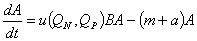(B.1a)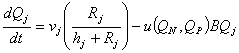(B.1b)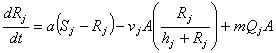(B.1c)

where j indicated nitrogen (N) or phosphorus (P).  In first equation, the net growth rate of plant biomass (A) was determined by gains due to cell production discounted by cell mortality due to two loss rates, death, m and dilution of the chemostat, a.  The producer birth rate depended on cellular nutrient quotas,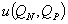.  In the simplest model, this function followed the standard Droop formulation (Grover 1997, Klausmeier et al. 2004):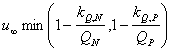(B.2)

where min(...) was the minimum of the arguments,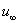was the physiologically maximal growth rate, kj was the minimal quota, and Qj was the nutrient content (quota).  In the Droop model, the plant's birth rate increased with nutrient quota of the plant in a saturating, hyperbolic fashion. The minimum function reflected the general belief that plant growth can be limited by only one macronutrient at a time (i.e., Leibig's Law of the Minimum; cf. Rhee 1974, 1978, and Klausmeier et al. 2004).  The true limiting nutrient had the smallest value of kj / Qj

Plant production was also a function of light.  Here, B is a Monod function for light, B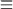L / (b + L), and represented the degree of light saturation of the producer; L was the incident light supply; and b was the half-saturation growth constant for light.  This representation assumed that light is an 'interactive-essential' resource with the limiting nutrient (following Tilman 1982, Huisman and Weissing 1995).  This representation meant that both the limiting nutrient and light co-limited the producer to some degree.  More mechanistic representations of light could have been used (e.g., Huisman and Weissing 1995), but we opted for this simplest form here.

Change in the tissue nutrient content of plants (Qj) reflected the balances between nutrient gains from nutrient uptake and nutrient losses due to “dilution by growth”.  The latter losses occurred as new biomass production used nutrients.  Uptake was represented by a Monod (saturating) function of available nutrient Rj, mediated by maximal uptake rate vj and half-saturation constant hj.  Free nutrient concentrations, in turn, followed a standard, open-system, chemostat form (Grover 1997, Klausmeier et al. 2004) where Sj was the concentration at which resource are supplied (at rate a), Rj was lost at rate a from the chemostat, algae took up Rj at rate v(Rj), and nutrients contained in dead algae were instantly recycled (at rate m).  Recycling of these nutrients in dead algae is not included in Klausmeier's (2004) model, but this difference between models proved qualitatively inconsequential for these analyses (not shown).

Model with Quota Saturation

In Fig. 4B, we studied how limitations to nutrient storage could accentuate deviations from the 1:1 relationship between N:P supply and N:P content of producers.  Adding limits to a producer's nutrient storage can shape response of plant stoichiometry to N:P supply. To incorporate this physiological constraint, we modified the production function, u(QP, QN) as follows (following Turpin 1988, Andersen 1997):(B.3)

where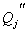were the maximum cell quotas for phosphorus or nitrogen.  In this modification, the plant production (birth) rate hyperbolically approachedas the cell quota of the most limiting nutrient Qj approached the.  The exact shape of this response further depended upon the difference between minimum and actual maximum quota,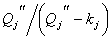, a term which accounted for physiological limits to nutrient storage (Turpin 1988, Andersen 1997).  In Fig. 4B, the value of nutrient quota of the producer was not allowed to exceed its maximum,.  Once this saturation point was reached, N:P ratio of the producer immediately plateaued to either its lowest point when N-limited,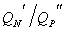, or to its highest point when P-limited,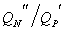, where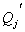was the realized (not absolute) minimum when nutrient j was limiting, given by:. (B.4)

It is obvious that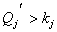and thatincreases as combined loss rate (m+a) increases.  This later result helped to explain why high loss rates accentuated the storage capacity effect (Fig. 4B).  Increased loss rate increased the numerator of the lower, N-limited plateau (, thus raising it) and increased the denominator of the upper plateau (, thus lowering it).

Table B1. Variables and parameters in the two-nutrient plant models.

 Symbol Units Description Value State variables A mg C/m3 Algal carbon (biomass) --- Qj mg/mg C Cell quota (content) of algae, nutrient j --- Rj mg/m3 Dissolved concentration of nutrient j --- t day Time --- Resource supply parameters L µmol photons·m-2·s-1 Incident light intensity 750 Sj mg/m3 Total supply of nutrient j 50,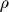SPmg N·(mg P)-1 N:P mass ratio of nutrient supply 1-50 Other parameters a day Dilution rate of chemostat --- b µmol photons·m-2·s-1 Half-saturation constant for light 36† B --- Degree of light saturation, L/(b+L) 0.95 hj mg/m3 Half saturation constant, nutrient j 0.7‡, 5‡ kj mg/mg C Minimum absolute quota of algae, nutrient j 0.004, 0.031‡ m day Added, density-independent losses of algae 0.05‡ Qj'' mg/mg C Maximum quota of algae, nutrient j 0.03, 0.122‡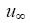day Growth rate at infinite quota 1.0‡ vj mg·(mg C)-1·d-1 Maximum uptake rate of algae for nutrient j 0.122§

Notes: Parameters listed for phosphorus (P), then nitrogen (N). Total loss rate of phytoplankton = m + a.

Source: Huisman et al. (1999).

Source: Andersen (1997).

§ Calculation assumes steady-state conditions (Grover 1997).

LITERATURE CITED

Andersen, T. 1997. Pelagic nutrient cycles: herbivores as sources and sinks. Springer-Verlag, New York, New York, USA.

Geider, R. J., H. L. MacIntyre, and T. M. Kana. 1998. A dynamic regulatory model of phytoplanktonic acclimation to light, nutrients, and temperature.  Limnology and Oceanography 43:679–694.

Grover, J. P. 1997. Resource competition. Chapman and Hall, New York, New York, USA.

Huisman, J., and F. J. Weissing. 1995. Competition for nutrients and light in a mixed water column: a theoretical analysis. American Naturalist 146:536–564.

Huisman, J., R. R. Jonker, C. Zonneveld, and F. J. Weissing. 1999. Competition for light between phytoplankton species: experimental tests of mechanistic theory. Ecology 80:211–222.

Legovic, T., and A. Cruzado. 1997. A model of phytoplankton growth on multiple nutrients based on the Michalis-Menton-Monod uptake, Droop's growth and Leibig's law. Ecological Modelling 99:19–31.

Rhee. G.-Y. 1974. Phosphate uptake under nitrate limitation by Scenedesmus sp. and its ecological implications. Journal of Phycology 10:470–475.

Turpin, D. H. 1988. Physiological mechanisms in phytoplankton resource competition. Pages 316–369  in C. D. Sandgren, editor. Growth and reproductive strategies of freshwater phytoplankton. Cambridge University Press, New York, New York, USA.

[Back to E086-100]